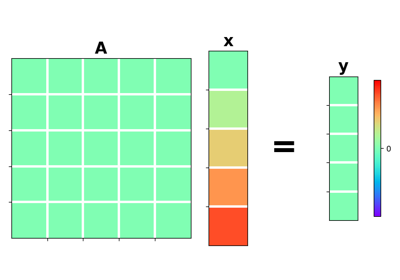# pylops.Zero#

class pylops.Zero(N, M=None, forceflat=None, dtype='float64', name='Z')[source]#

Zero operator.

Transform model into array of zeros of size $$N$$ in forward and transform data into array of zeros of size $$N$$ in adjoint.

Parameters
Nint or tuple

Number of samples in data (and model, if M is not provided). If a tuple is provided, this is interpreted as the data (and model) are nd-arrays.

Mint or tuple, optional

Number of samples in model. If a tuple is provided, this is interpreted as the model is an nd-array. Note that when M is a tuple, N must be also a tuple with the same number of elements.

forceflatbool, optional

New in version 2.2.0.

Force an array to be flattened after matvec and rmatvec. Note that this is only required when N and M are tuples (input and output arrays are nd-arrays).

dtypestr, optional

Type of elements in input array.

namestr, optional

New in version 2.0.0.

Name of operator (to be used by pylops.utils.describe.describe)

Notes

An Zero operator simply creates a null data vector $$\mathbf{y}$$ in forward mode:

$\mathbf{0} \mathbf{x} = \mathbf{0}_N$

and a null model vector $$\mathbf{x}$$ in forward mode:

$\mathbf{0} \mathbf{y} = \mathbf{0}_M$
Attributes
shapetuple

Operator shape

explicitbool

Operator contains a matrix that can be solved explicitly (True) or not (False)

Methods

 __init__(N[, M, forceflat, dtype, name]) adjoint() apply_columns(cols) Apply subset of columns of operator cond([uselobpcg]) Condition number of linear operator. conj() Complex conjugate operator div(y[, niter, densesolver]) Solve the linear problem $$\mathbf{y}=\mathbf{A}\mathbf{x}$$. dot(x) Matrix-matrix or matrix-vector multiplication. eigs([neigs, symmetric, niter, uselobpcg]) Most significant eigenvalues of linear operator. matmat(X) Matrix-matrix multiplication. matvec(x) Matrix-vector multiplication. reset_count() Reset counters rmatmat(X) Matrix-matrix multiplication. rmatvec(x) Adjoint matrix-vector multiplication. todense([backend]) Return dense matrix. toimag([forw, adj]) Imag operator toreal([forw, adj]) Real operator tosparse() Return sparse matrix. trace([neval, method, backend]) Trace of linear operator. transpose()

## Examples using pylops.Zero#Zero

Zero